Mechanics

# Newton’s second law

Newton ‘s second law , also known as the fundamental principle of dynamics , states that the resultant force acting on a body is equal to the product of its mass and acceleration . According to it, when a body is subjected to the action of a non-zero resultant force , that body will acquire an acceleration in the same direction and in the same direction as the resultant force.

## Newton’s second law

According to Newton’s second law, the acceleration obtained by a body is directly proportional to the resultant force applied on the body and also inversely proportional to the mass (inertia) of that body. In this sense, it is understood that, for a body to undergo changes in velocity, it is necessary that the forces acting on it do not cancel each other out.

In the following diagram, we show how it is possible to calculate the acceleration of the body, based on the magnitudes force and mass, in addition, it can be observed that the acceleration is given by the ratio between the change in velocity (ΔV) and a time interval (Δt):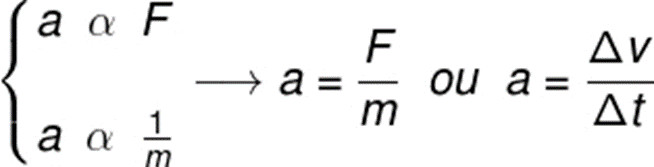The concept of resultant force is of great importance for understanding Newton’s second law. The resultant force is the vector sum of all forces acting on the same body. To do vector sums, it is necessary to take into account both the magnitude and the direction of the forces, thus: parallel forces are added, opposite forces are subtracted and perpendicular forces are added, according to the Pythagorean theorem .

## Newton’s second law formula

Newton’s second law formula is relatively simple, it indicates that the resultant force is equal to the product of the body’s mass and acceleration, check: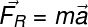R – resultant force (N)

m – body mass (kg)

a – acceleration (m/s²)

The following figure exemplifies situations in which, applying the same force, different accelerations are obtained, due to the great difference in the masses of the bodies, observe:

In addition to this form, Newton’s second law can be defined by other equations . Originally, it was written in terms of a physical quantity called momentum or momentum. According to this statement, the resultant force on a body is equal to the change in its momentum during a given time interval, check: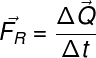ΔQ – variation of the momentum (kg.m/s)

Δt – time interval (s)

In the previous equation, Q represents the momentum of a body or system, which can be calculated using this equation: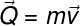Q – momentum (kg.m/s)

v – speed (m/s)

There is yet another alternative way of defining Newton’s second law. According to that description, the resultant force on a body can also be defined on the basis of the impulse applied to the body . Impulse, in turn, is a vector physical quantity , as well as variation in momentum ( ΔQ ), check: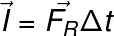In addition to the above formula, there is the impulse theorem . This theorem states that the impulse is equal to the application of a resultant force during an interval of time and produces a change in the momentum of a body or system of bodies, check:

## Examples of Newton’s second law

Check out some examples of everyday situations that help illustrate Newton’s second law:

• First, imagine pushing an empty shopping cart. Now, if this cart was full of goods and you applied the same force to it that you used while it was empty, would we have the same motion? No, since, with the cart full, its inertia will be greater, so it will be necessary to apply a greater force in order to obtain the same acceleration.
• In a tug of war, two groups of children compete, however, both apply the same force to the cable. In this case, the acceleration of the system will be zero, since the forces acting on it cancel each other out.
• When kicking a ball, it can be seen that the force applied by the kick defines the speed at which the ball will be thrown: the greater the force, the greater the acceleration acquired by the ball, the same applies to balls of different masses, since: the lighter the ball, the more acceleration it acquires.

Check out examples of solved exercises related to Newton’s second law:

Example 1) A body of mass 10 kg moves with a constant acceleration of 0.5 m/s². Determine the magnitude of the resultant force on this body.

Resolution:

Just multiply the mass of the body by the acceleration, check: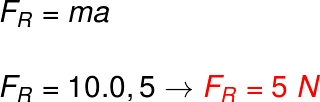Example 2) When subjected to a resultant force of 100 N, a body starts to move with a constant acceleration of 0.5 m/s². What is the mass of this body?

Resolution :

Let’s solve this exercise using Newton’s second law formula (F R = ma), note: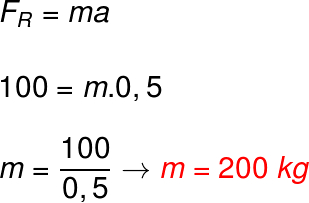When we apply the values ​​given by the statement in Newton’s second law formula, we find that the mass of the body must be 200 kg for it to develop an acceleration of 0.5 m/s².

## Exercises on Newton’s second law

Question 1) A 500 kg motorcycle is at rest and accelerates at a constant rate of 0.2 m/s² for a time interval of 5.0 seconds. Determine the magnitude of the force exerted on this motorcycle.

a) 250 N

b) 2500 N

c) 100 N

d) 100 N

e) 25 N

Template : letter c

Resolution:

To solve this exercise, just take into account the mass and acceleration of the body, observe:Question 2) A force of 200 N is applied to a body during a time interval of 2.0 s. The impulse exerted on this body during this time interval is equal to:

a) 400 Ns

b) 40 knots

c) 10 knots

d) 4000 Ns

e) 0.2 Ns

Template : letter a

Resolution :

The solution to this exercise requires the application of the impulse formula, check: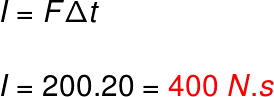When applying the exercise data, we find a thrust equal to 400 Ns, so the correct alternative is the letter a .